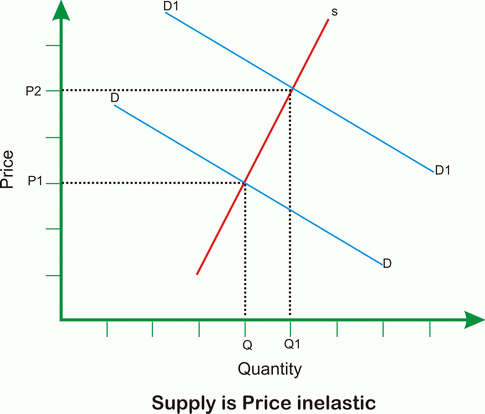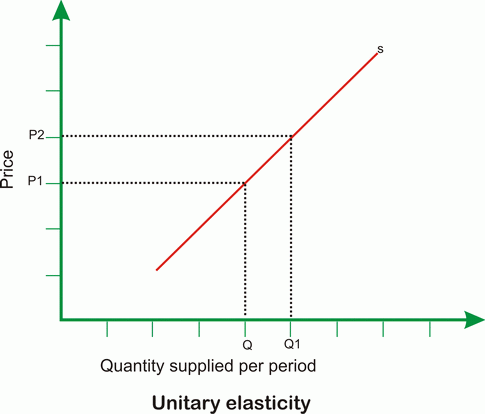# Price Elasticity of Supply

Price elasticity of supply is a measure of the responsiveness of quantity to a change in price.

In other words, it the percentage change in supply as compared to the percentage change in price of a commodity.

 Price Elasticity of Supply = % change in quantity Supplied % Change in price

Elasticity = 0: if the supply curve is vertical, and there is no response to prices.

Elasticity = ?: if the supply curve is horizontal.

Supply is price elastic if the price elasticity of supply is greater than 1, unit price elastic if it is equal to 1, and price inelastic if it is less than 1.

Supply is Price Elastic when the percentage change in quantity supplied is more than the percentage change in Price of the comoditity. PES is more than 1.Supply is Price Inelastic when the percentage change in quantity supplied is less than the percentage change in Price of the comoditity. PES is less than 1.## Types of Price elasticity of Supply

### Unitary Price Elasticity of Supply### Perfectly price elasticity of supply### Infinite price elasticity of Supply